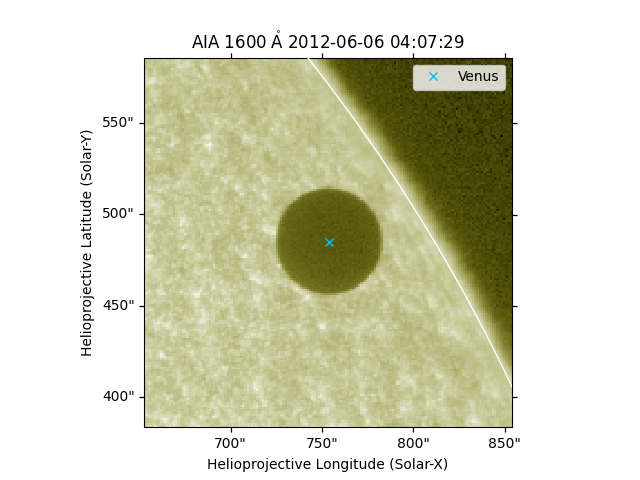# Overplotting the position of the Venus transit¶

How to accurately plot the position of Venus as it transitted in front of the Sun as observed by SDO/AIA.

```import matplotlib.pyplot as plt

import astropy.units as u
from astropy.coordinates import SkyCoord
from astropy.coordinates import solar_system_ephemeris

import sunpy.map
from sunpy.coordinates import get_body_heliographic_stonyhurst
```

```f = download_file('http://jsoc.stanford.edu/data/events/Venus_AIA24s_1600/Out/fits/20120606_040731_UTC.0041.fits')
aiamap = sunpy.map.Map(f)
```

For this example, we require high precision ephemeris information. The built-in ephemeris provided by astropy are not accurate enough. This requires `jplephem` to be installed. This will also trigger a download of about ~10 MB.

```solar_system_ephemeris.set('de432s')
```

Now we get the position of venus and convert it into the SDO/AIA coordinates.

```venus = get_body_heliographic_stonyhurst('venus', aiamap.date, observer=aiamap.observer_coordinate)
venus_hpc = venus.transform_to(aiamap.coordinate_frame)
```

Out:

```INFO: Apparent body location accounts for 144.14 seconds of light travel time [sunpy.coordinates.ephemeris]
```

Let’s crop the image with Venus at its center.

```fov = 100 * u.arcsec
top_right = SkyCoord(venus_hpc.Tx + fov, venus_hpc.Ty + fov, frame=aiamap.coordinate_frame)
bottom_left = SkyCoord(venus_hpc.Tx - fov, venus_hpc.Ty - fov, frame=aiamap.coordinate_frame)
smap = aiamap.submap(top_right, bottom_left)
```

Let’s plot the results.

```ax = plt.subplot(projection=smap)
smap.plot()
smap.draw_limb()
ax.plot_coord(venus_hpc, 'x', color='white')
plt.show()
```Total running time of the script: ( 0 minutes 3.928 seconds)

Gallery generated by Sphinx-Gallery